# Introduction

## Act like a Crowd Counting Scientist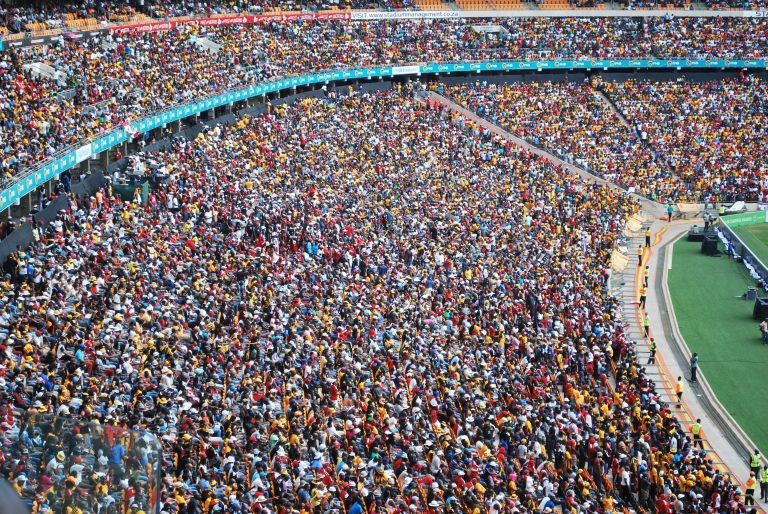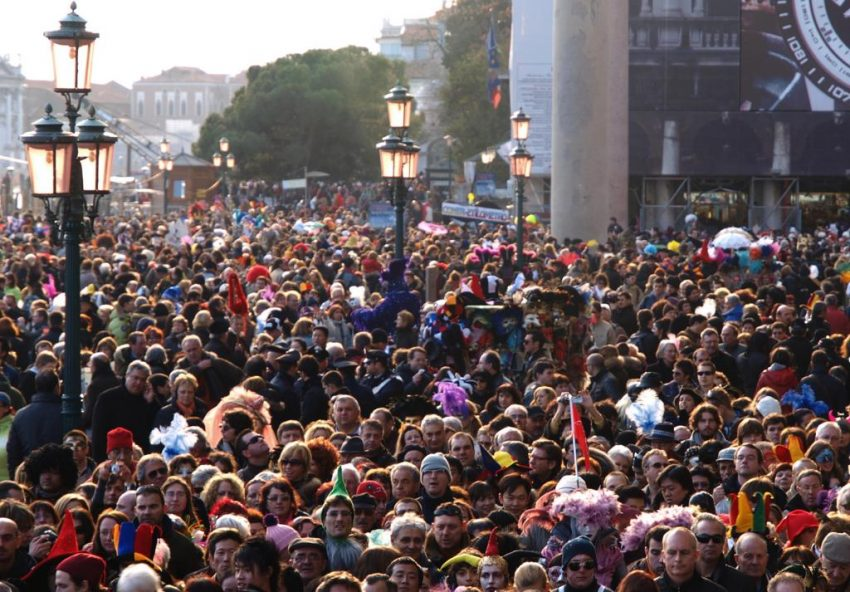• 什么是人群计数？
• 为什么需要人群计数？
• 了解用于人群计数的不同计算机视觉技术
• CSRNet的架构和培训方法
• 在Python中构建自己的Crowd Counting模型

## What is Crowd Counting?# Why is Crowd Counting useful?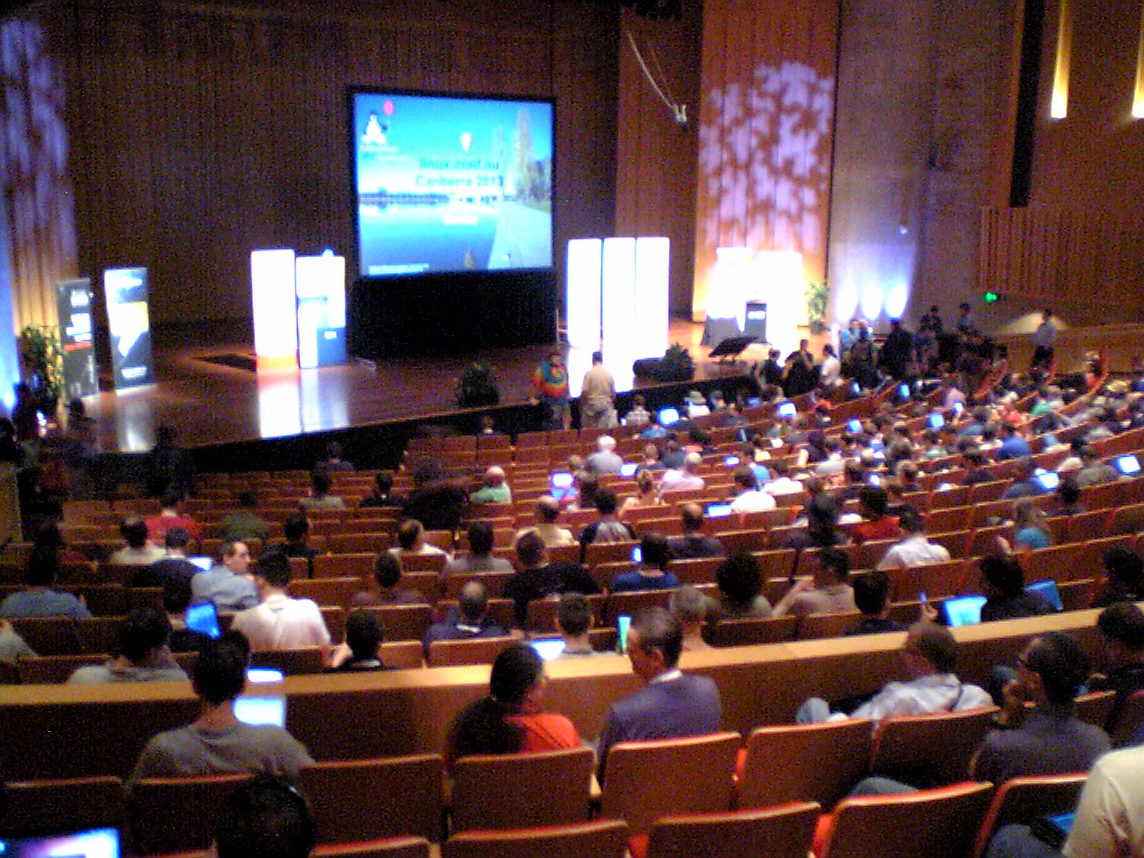• 计算参加体育赛事的人数
• 估计有多少人参加了就职典礼或游行（也许是政治集会）
• 监测人流量大的区域
• 帮助配置人员配置和资源分配

## Understanding the Different Computer Vision Techniques for Crowd Counting

### 4.基于CNN的方法

CSRNet是我们将在本文中实现的一种技术，它可以部署更深入的CNN，用于捕获高级功能并生成高质量的密度映射，而不会扩展网络复杂性。在跳转到编码部分之前，让我们了解CSRNet是什么。

# 了解CSRNet的架构和培训方法

CSRNet使用VGG-16作为前端，因为它具有强大的转移学习能力。 VGG的输出大小是原始输入大小的1/3。 CSRNet还在后端使用扩张的卷积层。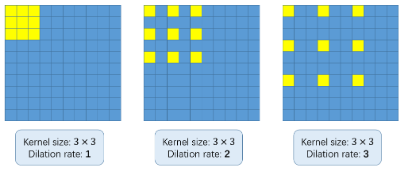### 基础数学（推荐，但可选）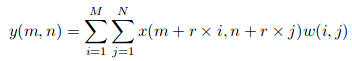（[k +（k-1）（r-1）] * [k +（k-1）（r-1）]）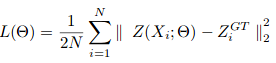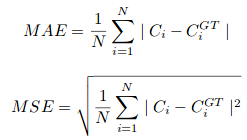Here, Ci is the estimated count: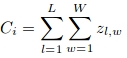L和W是预测密度图的宽度。

## 建立自己的人群计数模型

git clone https://github.com/leeyeehoo/CSRNet-pytorch.git


# importing libraries
import h5py
import scipy.io as io
import PIL.Image as Image
import numpy as np
import os
import glob
from matplotlib import pyplot as plt
from scipy.ndimage.filters import gaussian_filter
import scipy
import json
from matplotlib import cm as CM
from image import *
from model import CSRNet
import torch
from tqdm import tqdm
%matplotlib inline

# function to create density maps for images
def gaussian_filter_density(gt):
print (gt.shape)
density = np.zeros(gt.shape, dtype=np.float32)
gt_count = np.count_nonzero(gt)
if gt_count == 0:
return density

pts = np.array(list(zip(np.nonzero(gt), np.nonzero(gt))))
leafsize = 2048
# build kdtree
tree = scipy.spatial.KDTree(pts.copy(), leafsize=leafsize)
# query kdtree
distances, locations = tree.query(pts, k=4)

print ('generate density...')
for i, pt in enumerate(pts):
pt2d = np.zeros(gt.shape, dtype=np.float32)
pt2d[pt,pt] = 1.
if gt_count &gt; 1:
sigma = (distances[i]+distances[i]+distances[i])*0.1
else:
sigma = np.average(np.array(gt.shape))/2./2. #case: 1 point
density += scipy.ndimage.filters.gaussian_filter(pt2d, sigma, mode='constant')
print ('done.')
return density

#setting the root to the Shanghai dataset you have downloaded
# change the root path as per your location of dataset
root = '/home/pulkit/CSRNet-pytorch/'


part_A_train = os.path.join(root,'part_A/train_data','images')
part_A_test = os.path.join(root,'part_A/test_data','images')
part_B_train = os.path.join(root,'part_B/train_data','images')
part_B_test = os.path.join(root,'part_B/test_data','images')
path_sets = [part_A_train,part_A_test]

img_paths = []
for path in path_sets:
for img_path in glob.glob(os.path.join(path, '*.jpg')):
img_paths.append(img_path)

for img_path in img_paths:
print (img_path)
k = np.zeros((img.shape,img.shape))
gt = mat["image_info"][0,0][0,0]
for i in range(0,len(gt)):
if int(gt[i])<img.shape and int(gt[i])<img.shape:
k[int(gt[i]),int(gt[i])]=1
k = gaussian_filter_density(k)
with h5py.File(img_path.replace('.jpg','.h5').replace('images','ground-truth'), 'w') as hf:
hf['density'] = k


plt.imshow(Image.open(img_paths))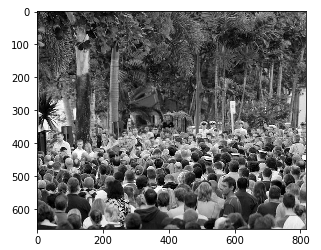gt_file = h5py.File(img_paths.replace('.jpg','.h5').replace('images','ground-truth'),'r')
groundtruth = np.asarray(gt_file['density'])
plt.imshow(groundtruth,cmap=CM.jet)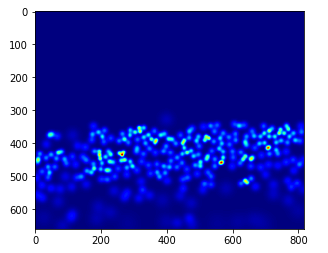np.sum(groundtruth)


## 270.32568

path_sets = [part_B_train,part_B_test]
img_paths = []
for path in path_sets:
for img_path in glob.glob(os.path.join(path, '*.jpg')):
img_paths.append(img_path)

# creating density map for part_b images

for img_path in img_paths:
print (img_path)
k = np.zeros((img.shape,img.shape))
gt = mat["image_info"][0,0][0,0]
for i in range(0,len(gt)):
if int(gt[i])<img.shape and int(gt[i])<img.shape:
k[int(gt[i]),int(gt[i])]=1
k = gaussian_filter_density(k)
with h5py.File(img_path.replace('.jpg','.h5').replace('images','ground-truth'), 'w') as hf:
hf['density'] = k


• 在model.py中，将第18行中的xrange更改为range
• 更改model.py中的第19行：list(self.frontend.state_dict().items())[i].data[:] = list(mod.state_dict().items())[i].data[:]
• 在image.py中，将ground_truth替换为ground-true

cd CSRNet-pytorch
python train.py part_A_train.json part_A_val.json 0 0


#importing libraries
import h5py
import scipy.io as io
import PIL.Image as Image
import numpy as np
import os
import glob
from matplotlib import pyplot as plt
from scipy.ndimage.filters import gaussian_filter
import scipy
import json
import torchvision.transforms.functional as F
from matplotlib import cm as CM
from image import *
from model import CSRNet
import torch
%matplotlib inline

from torchvision import datasets, transforms
transform=transforms.Compose([
transforms.ToTensor(),transforms.Normalize(mean=[0.485, 0.456, 0.406],
std=[0.229, 0.224, 0.225]),
])

#defining the location of dataset
root = '/home/pulkit/CSRNet/ShanghaiTech/CSRNet-pytorch/'
part_A_train = os.path.join(root,'part_A/train_data','images')
part_A_test = os.path.join(root,'part_A/test_data','images')
part_B_train = os.path.join(root,'part_B/train_data','images')
part_B_test = os.path.join(root,'part_B/test_data','images')
path_sets = [part_A_test]

#defining the image path
img_paths = []
for path in path_sets:
for img_path in glob.glob(os.path.join(path, '*.jpg')):
img_paths.append(img_path)

model = CSRNet()

#defining the model
model = model.cuda()

#loading the trained weights


mae = 0
for i in tqdm(range(len(img_paths))):
img = transform(Image.open(img_paths[i]).convert('RGB')).cuda()
gt_file = h5py.File(img_paths[i].replace('.jpg','.h5').replace('images','ground-truth'),'r')
groundtruth = np.asarray(gt_file['density'])
output = model(img.unsqueeze(0))
mae += abs(output.detach().cpu().sum().numpy()-np.sum(groundtruth))
print (mae/len(img_paths))


from matplotlib import cm as c
img = transform(Image.open('part_A/test_data/images/IMG_100.jpg').convert('RGB')).cuda()

output = model(img.unsqueeze(0))
print("Predicted Count : ",int(output.detach().cpu().sum().numpy()))
temp = np.asarray(output.detach().cpu().reshape(output.detach().cpu().shape,output.detach().cpu().shape))
plt.imshow(temp,cmap = c.jet)
plt.show()
temp = h5py.File('part_A/test_data/ground-truth/IMG_100.h5', 'r')
temp_1 = np.asarray(temp['density'])
plt.imshow(temp_1,cmap = c.jet)
print("Original Count : ",int(np.sum(temp_1)) + 1)
plt.show()
print("Original Image")
plt.show()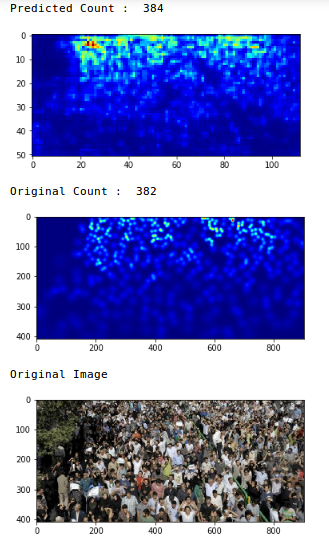# End Notes

05-15636

#### C3F：首个开源人群计数算法框架

01-137330

#### 人群计数：人群计数研究综述（2018.11）12-031万+

#### 不可不知的七大统计模型

12-254423

#### 人群密度估计 - CVPR2018最新论文CSRNet原理、配套代码解析

06-05367

#### CrowdNet介绍：（人群计数）

03-053573

#### CNN深度学习——人群计数

03-227万+

#### 男生更看重女生的身材脸蛋，还是思想？

03-2514万+

#### 当HR压你价，说你只值7K，你该怎么回答？

06-101303

#### 基于多列卷积神经网络的单图像人群计数（人群计数）

10-106435

#### 人群分析、人群计数 开源代码文献及数据库©️2020 CSDN 皮肤主题: 大白 设计师: CSDN官方博客点击重新获取扫码支付1.余额是钱包充值的虚拟货币，按照1:1的比例进行支付金额的抵扣。
2.余额无法直接购买下载，可以购买VIP、C币套餐、付费专栏及课程。余额充值